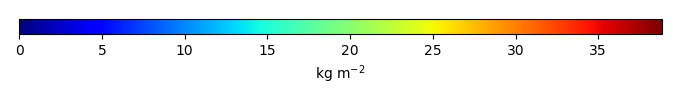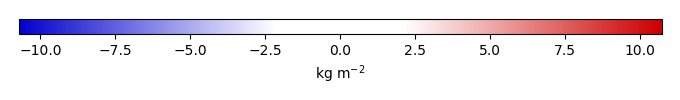# Mean State

Period Mean (original grids) [Pg]
Model Period Mean (intersection) [Pg]
Model Period Mean (complement) [Pg]
Benchmark Period Mean (intersection) [Pg]
Benchmark Period Mean (complement) [Pg]
Bias [kg m-2]
Bias Score 
Spatial Distribution Score 
Overall Score 
Benchmark [-] 38.7
CLM4 [-] 58.3 59.2 0.192 38.7 0.0457 4.86 0.544 0.700 0.622
CLM4.5 [-] 58.7 58.9 0.216 38.7 0.0457 4.82 0.458 0.593 0.525
CLM5 [-] 42.9 43.3 0.201 38.7 0.0457 1.34 0.709 0.904 0.807
Period Mean (original grids) [Pg]
Model Period Mean (intersection) [Pg]
Model Period Mean (complement) [Pg]
Benchmark Period Mean (intersection) [Pg]
Benchmark Period Mean (complement) [Pg]
Bias [kg m-2]
Bias Score 
Spatial Distribution Score 
Overall Score 
Benchmark [-] 11.9
CLM4 [-] 7.47 6.59 0.00842 11.9 0.0196 -0.786 0.554 0.853 0.704
CLM4.5 [-] 2.44 2.14 0.0206 11.9 0.0196 -1.46 0.429 0.524 0.476
CLM5 [-] 3.54 3.10 0.0369 11.9 0.0196 -1.31 0.465 0.623 0.544
Period Mean (original grids) [Pg]
Model Period Mean (intersection) [Pg]
Model Period Mean (complement) [Pg]
Benchmark Period Mean (intersection) [Pg]
Benchmark Period Mean (complement) [Pg]
Bias [kg m-2]
Bias Score 
Spatial Distribution Score 
Overall Score 
Benchmark [-] 24.6
CLM4 [-] 13.7 13.1 0.259 24.5 0.0612 -1.15 0.503 0.753 0.628
CLM4.5 [-] 6.79 6.36 0.272 24.5 0.0612 -1.85 0.456 0.768 0.612
CLM5 [-] 17.0 16.3 0.393 24.5 0.0612 -0.721 0.558 0.788 0.673
Period Mean (original grids) [Pg]
Model Period Mean (intersection) [Pg]
Model Period Mean (complement) [Pg]
Benchmark Period Mean (intersection) [Pg]
Benchmark Period Mean (complement) [Pg]
Bias [kg m-2]
Bias Score 
Spatial Distribution Score 
Overall Score 
Benchmark [-] 36.8
CLM4 [-] 79.6 75.8 3.45 35.7 1.10 19.6 0.314 0.586 0.450
CLM4.5 [-] 66.7 63.5 2.96 35.7 1.10 15.1 0.405 0.836 0.620
CLM5 [-] 49.4 46.8 2.28 35.7 1.10 8.69 0.616 0.935 0.776
Period Mean (original grids) [Pg]
Model Period Mean (intersection) [Pg]
Model Period Mean (complement) [Pg]
Benchmark Period Mean (intersection) [Pg]
Benchmark Period Mean (complement) [Pg]
Bias [kg m-2]
Bias Score 
Spatial Distribution Score 
Overall Score 
Benchmark [-] 38.4
CLM4 [-] 45.4 33.4 12.7 38.3 0.0891 -0.0468 0.565 0.569 0.567
CLM4.5 [-] 44.2 31.5 13.2 38.3 0.0891 -0.330 0.499 0.547 0.523
CLM5 [-] 36.1 23.9 13.0 38.3 0.0891 -1.35 0.543 0.685 0.614
Period Mean (original grids) [Pg]
Model Period Mean (intersection) [Pg]
Model Period Mean (complement) [Pg]
Benchmark Period Mean (intersection) [Pg]
Benchmark Period Mean (complement) [Pg]
Bias [kg m-2]
Bias Score 
Spatial Distribution Score 
Overall Score 
Benchmark [-] 43.7
CLM4 [-] 30.3 28.7 0.664 43.4 0.257 -1.07 0.606 0.806 0.706
CLM4.5 [-] 25.6 24.0 0.704 43.4 0.257 -1.62 0.504 0.722 0.613
CLM5 [-] 25.4 24.0 0.749 43.4 0.257 -1.74 0.587 0.855 0.721
Period Mean (original grids) [Pg]
Model Period Mean (intersection) [Pg]
Model Period Mean (complement) [Pg]
Benchmark Period Mean (intersection) [Pg]
Benchmark Period Mean (complement) [Pg]
Bias [kg m-2]
Bias Score 
Spatial Distribution Score 
Overall Score 
Benchmark [-] 13.7
CLM4 [-] 7.52 1.46 5.92 13.5 0.139 -1.87 0.410 0.621 0.515
CLM4.5 [-] 7.56 1.67 5.76 13.5 0.139 -1.82 0.416 0.655 0.535
CLM5 [-] 11.5 4.26 7.02 13.5 0.139 -1.29 0.463 0.617 0.540
Period Mean (original grids) [Pg]
Model Period Mean (intersection) [Pg]
Model Period Mean (complement) [Pg]
Benchmark Period Mean (intersection) [Pg]
Benchmark Period Mean (complement) [Pg]
Bias [kg m-2]
Bias Score 
Spatial Distribution Score 
Overall Score 
Benchmark [-] 352.
CLM4 [-] 582. 459. 123. 348. 3.81 2.37 0.473 0.480 0.476
CLM4.5 [-] 559. 404. 156. 348. 3.81 1.57 0.458 0.565 0.512
CLM5 [-] 589. 355. 233. 348. 3.81 0.826 0.590 0.766 0.678
Period Mean (original grids) [Pg]
Model Period Mean (intersection) [Pg]
Model Period Mean (complement) [Pg]
Benchmark Period Mean (intersection) [Pg]
Benchmark Period Mean (complement) [Pg]
Bias [kg m-2]
Bias Score 
Spatial Distribution Score 
Overall Score 
Benchmark [-] 2.53
CLM4 [-] 1.35 0.110 1.23 2.53 0.00250 -1.09 0.384 0.0106 0.197
CLM4.5 [-] 1.53 0.121 1.41 2.53 0.00250 -1.09 0.385 0.0170 0.201
CLM5 [-] 1.68 0.229 1.44 2.53 0.00250 -1.04 0.396 0.0230 0.210
Period Mean (original grids) [Pg]
Model Period Mean (intersection) [Pg]
Model Period Mean (complement) [Pg]
Benchmark Period Mean (intersection) [Pg]
Benchmark Period Mean (complement) [Pg]
Bias [kg m-2]
Bias Score 
Spatial Distribution Score 
Overall Score 
Benchmark [-] 11.1
CLM4 [-] 5.39 4.73 0.362 10.9 0.149 -1.15 0.453 0.572 0.512
CLM4.5 [-] 11.4 10.6 0.532 10.9 0.149 1.23 0.509 0.581 0.545
CLM5 [-] 11.9 11.0 0.602 10.9 0.149 1.49 0.573 0.684 0.628
Period Mean (original grids) [Pg]
Model Period Mean (intersection) [Pg]
Model Period Mean (complement) [Pg]
Benchmark Period Mean (intersection) [Pg]
Benchmark Period Mean (complement) [Pg]
Bias [kg m-2]
Bias Score 
Spatial Distribution Score 
Overall Score 
Benchmark [-] 1.55
CLM4 [-] 16.4 3.22 13.1 1.52 0.0247 2.94 0.543 0.541 0.542
CLM4.5 [-] 20.4 3.67 16.5 1.52 0.0247 3.74 0.494 0.471 0.482
CLM5 [-] 17.7 3.58 14.0 1.52 0.0247 3.61 0.472 0.490 0.481
Period Mean (original grids) [Pg]
Model Period Mean (intersection) [Pg]
Model Period Mean (complement) [Pg]
Benchmark Period Mean (intersection) [Pg]
Benchmark Period Mean (complement) [Pg]
Bias [kg m-2]
Bias Score 
Spatial Distribution Score 
Overall Score 
Benchmark [-] 0.219
CLM4 [-] 5.10 0.0841 5.06 0.219 -0.442 0.463 0.173 0.318
CLM4.5 [-] 3.41 0.0679 3.39 0.219 -0.490 0.446 0.133 0.289
CLM5 [-] 3.48 0.0759 3.42 0.219 -0.459 0.463 0.0991 0.281
Period Mean (original grids) [Pg]
Model Period Mean (intersection) [Pg]
Model Period Mean (complement) [Pg]
Benchmark Period Mean (intersection) [Pg]
Benchmark Period Mean (complement) [Pg]
Bias [kg m-2]
Bias Score 
Spatial Distribution Score 
Overall Score 
Benchmark [-] 100.
CLM4 [-] 208. 207. 0.431 100. 0.0690 13.1 0.393 0.385 0.389
CLM4.5 [-] 181. 180. 0.479 100. 0.0690 9.91 0.436 0.554 0.495
CLM5 [-] 152. 151. 0.453 100. 0.0690 6.40 0.602 0.843 0.722
Period Mean (original grids) [Pg]
Model Period Mean (intersection) [Pg]
Model Period Mean (complement) [Pg]
Benchmark Period Mean (intersection) [Pg]
Benchmark Period Mean (complement) [Pg]
Bias [kg m-2]
Bias Score 
Spatial Distribution Score 
Overall Score 
Benchmark [-] 18.4
CLM4 [-] 20.1 20.3 0.146 18.4 0.0281 0.485 0.529 0.448 0.489
CLM4.5 [-] 15.4 15.3 0.235 18.4 0.0281 -0.252 0.528 0.485 0.507
CLM5 [-] 21.7 21.7 0.382 18.4 0.0281 0.759 0.617 0.725 0.671
Period Mean (original grids) [Pg]
Model Period Mean (intersection) [Pg]
Model Period Mean (complement) [Pg]
Benchmark Period Mean (intersection) [Pg]
Benchmark Period Mean (complement) [Pg]
Bias [kg m-2]
Bias Score 
Spatial Distribution Score 
Overall Score 
Benchmark [-] 7.28
CLM4 [-] 3.86 4.08 0.0995 7.15 0.128 -0.427 0.488 0.519 0.504
CLM4.5 [-] 4.36 4.41 0.104 7.15 0.128 -0.255 0.465 0.523 0.494
CLM5 [-] 5.25 5.14 0.150 7.15 0.128 0.169 0.530 0.533 0.532

# Temporally integrated period mean

BENCHMARK MEANMODEL MEANBIASBIAS SCORESPATIAL TAYLOR DIAGRAMMODEL COLORS# Spatially integrated regional mean

MODEL COLORS# All Models

BenchmarkCLM4CLM4.5CLM5# Data Information

title: Global Tropical Forest Biomass

institution: NASA-JPL (NASA Jet Propulsion Laboratory, California Institute of Technology, Pasadena, California 91109, USA)

institute_id: NASA-JPL

source: 4079 in situ inventory plots, satellite light detection and ranging (LiDAR) samples of forest structure, and optical and microwave imagery (1-km resolution) to extrapolate estimates

history: 2015-01-08-T06:37:41Z Converted from 1 km x 1 km to 0.5 degree x 0.5 degree by Mingquan Mu (mmu@uci.edu) on Fri Jan 9 10:37:41 PST 2015

creation_date: 2015-01-08-T06:37:41Z

Conventions: CF-1.6

product: observations

data_structure: grid

realm: land

contact: Prof. James T. Randerson (jranders@uci.edu) or Dr. Mingquan Mu (mmu@uci.edu)

references: Saatchi, S. S., N. L. Harris, S. Brown, M. Lefsky, E. T. A. Mitchard, W. Salas, B. R. Zutta, W. Buermann, S. L. Lewis, S. Hagen, S. Petrova, L. White, M. Silman, and A. Morel (2011), Benchmark map of forest carbon stocks in tropical regions across three continents, Proc. Nat. Acad. Sci., 108(24):9899-9904, doi:10.1073/pnas.1019576108.